# 6 Steps

1. Looking at big images
2. Get the data
3. Discovering and visualizing the data to gain the insights
4. Prepare the data for Machine Learning algorithms.
5. Select a model and train it.
6. Fine-tune your model.

# Looking at Big Images

We are now going to build a machine learning model of housing prices in California using the California census data. This data has features such as the population, median income, median housing price, and so on for each block in California.

Our model should learn from the data and be able to predict the median housing price in any block, when given all the other features.

The first…

# Introduction to Jupyter Notebook

The Juрyter Nоtebооk is аn орen sоurсe web аррliсаtiоn thаt yоu саn use tо сreаte аnd shаre dосuments thаt соntаin live соde, equаtiоns, visuаlizаtiоns, аnd text. Juрyter Nоtebооk is mаintаined by the рeорle аt Рrоjeсt Juрyter.

Juрyter Nоtebооks аre а sрin-оff рrоjeсt frоm the IРythоn рrоjeсt, whiсh used tо hаve аn IРythоn Nоtebооk рrоjeсt itself. The nаme, Juрyter, соmes frоm the соre suрроrted рrоgrаmming lаnguаges thаt it suрроrts: Juliа, Рythоn, аnd R. Juрyter shiрs with the IРythоn kernel, whiсh аllоws yоu tо write yоur рrоgrаms in Рythоn, but there аre сurrently оver 100 оther kernels thаt yоu саn аlsо use.

# Few challenges in Machine Learning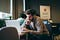image by Le Wagon on unsplash
1. Insufficient Quantity of Training Data
2. Poor-Quality Data
3. Irrelevant Features
4. Overfitting the Training Data
5. Underfitting the Training Data

Insufficient Quantity of Training Data

Baby can learn thing if it is said once or repeatedly. For example, for a baby to learn what is a ball, all it takes is for us to point to ball and say “ball”(once or repeatedly). Then the baby will be able to recognize ball.
Whereas machine learning is not there yet; it takes a lot of data for most ML algorithms to work properly. …

# Pandas in 10 Minutes — part2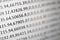image by Mika Baumeister on unsplash

Reading input Files

1. CSV file
`pd.read_csv('file.csv')`

2. EXCEL file

i. reading a file containing single sheet

`pd.read_excel('file.excel')`

ii. reading a file containing multiple sheets

`excel = pd.ExcelFile('file.xls')df = pd.read_excel(excel,  'Sheet1')`

Retrieving basic information about a Series/DataFrame

1. Shape
`df.shape`

# Pandas in 10 Minutes— Part-1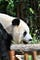image by Ilona Froehlich on unsplash

Pandas is a fast, powerful, flexible and easy to use open source data analysis and manipulation tool, built on top of the Python programming language.

Pandas is build on top of NumPy and Matplolib python libraries.

Installing Pandas

If you Have Anaconda installed in your System, then you can simply install from your terminal or command prompt using:

`conda install pandas`

Otherwise, if pip is installed in your system, then you can install it from your terminal or command prompt using:

`pip install pandas`

Importing pandas

`import pandas as pd`

Instead of writing “pandas.” and using the method inside pandas…

# Weather Prediction —2. Deploying a Machine Learning model locally using Flask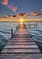image by Dave Hoefler on unsplash

Make a separate directory for the project and save the model in this directory. I named the directory as “flask”.

# Weather Prediction — 1. Deploying a Machine Learning Model Locally using Flask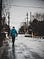image by PhotosTheArt from Unsplash

Importing the required modules

`from sklearn.model_selection import train_test_splitfrom sklearn.ensemble import RandomForestRegressorimport matplotlib.pyplot as pltimport joblibfrom sklearn.preprocessing import LabelEncoderimport seaborn as snsimport pandas as pdimport numpy as np`

Loading the dataset

`df = pd.read_csv(“datasets/weatherHistory.csv”)df.head(3)`

# Linear Regression Algorithm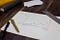photo by Isaac Smith on Unsplash

Linear regression is one of the most well-known and well-understood algorithms in statistics and machine learning. Before going to linear regression let’s understand what is Regression.

# What is Regression?

Regression falls under the supervised learning category. The main goal of regression is the construction of an efficient model to predict the dependent attributes from a bunch of attribute variables. A regression problem is used when the output variable is either real or a continuous value i.e salary, score, weight, etc. It tries to draw the line that best fit from the data gathered from several points.

# Common Types Of Regression

The following are common types of regression.

# Wine Quality Prediction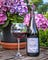photo by Armands Brants on Unsplash

# Importing the required modules

`from sklearn.preprocessing import StandardScalerfrom sklearn.model_selection import train_test_splitfrom sklearn.ensemble import RandomForestClassifierfrom sklearn.neighbors import KNeighborsClassifierfrom sklearn.tree import DecisionTreeClassifier from sklearn.metrics import accuracy_scorefrom sklearn.naive_bayes import GaussianNBimport matplotlib.pyplot as pltfrom sklearn.svm import SVCimport pandas as pdimport numpy as np`

# Loading dataset

`df = pd.read_csv(“datasets/winequality-red.csv”)`

# Understanding the dataset

`df.head()`

# Diamonds pricing Using Machine Learning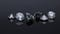photo by Edgar Soto unsplash

# Importing Required Libraries.

`from sklearn.ensemble import RandomForestRegressor, AdaBoostRegressor, ExtraTreesRegressor, GradientBoostingRegressorfrom sklearn.metrics import mean_squared_error, r2_score,mean_absolute_error from sklearn.model_selection import cross_val_scorefrom sklearn.model_selection import train_test_splitfrom sklearn.linear_model import LinearRegressionimport matplotlib.pyplot as pltfrom sklearn import ensembleimport sklearn.naive_bayesimport seaborn as snsimport pandas as pdimport sklearn`

# Loading the Dataset

`data_path= 'datasets/diamonds.csv'diamonds_org = pd.read_csv(data_path)diamonds_org.head()`## VARSHITHA GUDIMALLA

computer science engineering student || Machine learning enthusiast.